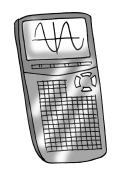### Home > PC3 > Chapter Ch8 > Lesson 8.2.1 > Problem8-61

8-61.

There are no buttons for secant, cosecant, or cotangent on a calculator. In order to evaluate these expressions, the definition of the reciprocal function needs to be utilized. Use a calculator to evaluate each of the expressions below. The first answer is given as a check. All angles are in radians.1. $\operatorname { csc } ( 3 ) \approx 7.086$

1. $\cot\left(\frac{2}{7}\right)$

$\frac{1}{\tan\left(\frac{2}{7}\right)}$

1. $\sec\left(\frac{4\pi}{5}\right)$

$\frac{1}{\cos\left(\frac{4\pi}{5}\right)}$